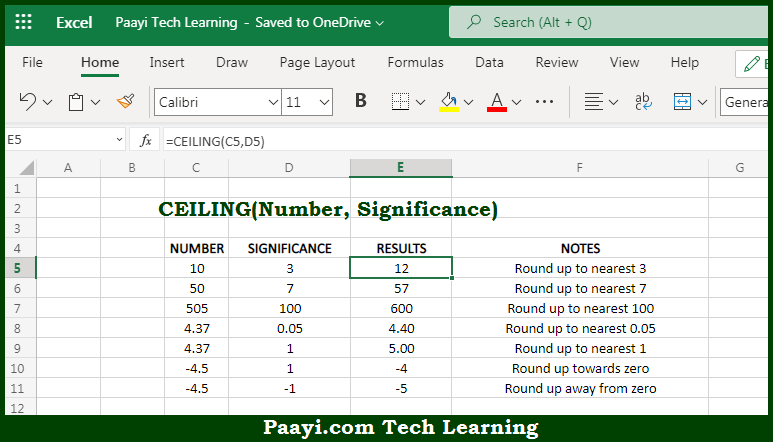# Learn How to Use Microsoft Excel CEILING Function

Written by | 0 Comments | 617 Views

In this article, you will learn how to use the Microsoft Excel CEILING function and its prime function in Microsoft Excel. You will also get to know the Microsoft Excel CEILING function return value and syntax with the help of some examples.

Microsoft Excel CEILING Function

The main function of the Microsoft Excel CEILING function is to round up a number to the nearest multiple. So, with the help of the CEILING function, you can able to round up the number to the nearest specified multiple. The function CEILING function is same as the MROUND function, but the CEILING function always rounds up. So, with the help of the Microsoft Excel CEILING function, you can easily round up the number to the nearest multiple.

Return Value of CEILING Function

The return value will be the rounded number.

Syntax of CEILING Function

=CEILING(number, significance)

Where the arguments:

• number: This is the number you want to round up.
• significance: This is the multiple used while rounding up the number.

## How to Use Microsoft Excel CEILING Function?Microsoft Excel CEILING function is used to round up a number up to a given multiple. The multiple to use for rounding is provided as the argument while using the function. If the number is already an exact multiple, no rounding occurs and the original number is returned. The function CEILING function same as the MROUND function, but the CEILING function always rounds up. So, with the help of the Microsoft Excel CEILING function, you can easily round up the number to the nearest multiple.